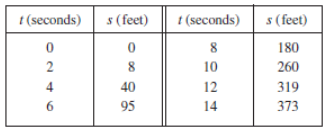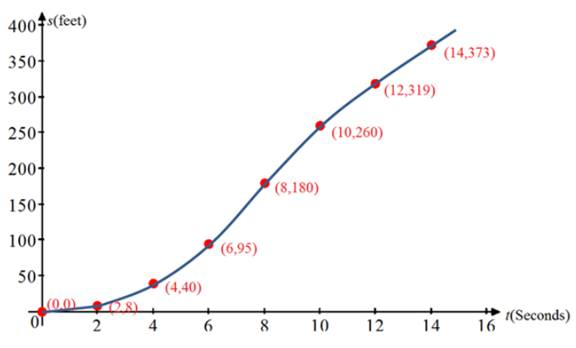# Thespeed after 6 seconds.### Single Variable Calculus: Concepts...

4th Edition
James Stewart
Publisher: Cengage Learning
ISBN: 9781337687805### Single Variable Calculus: Concepts...

4th Edition
James Stewart
Publisher: Cengage Learning
ISBN: 9781337687805

#### Solutions

Chapter 2, Problem 47RE

(a)

To determine

## To find:Thespeed after 6 seconds.

Expert Solution

The velocity (speed) after 6 seconds is about 35ft/sec.

### Explanation of Solution

Given information:

The car starts from rest and its distance traveled is recorded in following table.Figure (1)

Calculation:

For time period [0,2] .

Averagevelocity=changeinthepositionchangeinthetime=8020=82=4

For time period [2,4] .

Averagevelocity=changeinthepositionchangeinthetime=40842=322=16

For time period [4,6] .

Averagevelocity=changeinthepositionchangeinthetime=954064=552=27.5

For time period [6,8] .

Averagevelocity=changeinthepositionchangeinthetime=1809564=852=42.5

The slope of the secant line from t=4 to t=6 be 27.5ft/sec.

The slope of the secant line from t=6 to t=8 be 42.5ft/sec.

So, the velocity at t=6 would be exactly half way between 27.5ft/sec. and 42.5ft/sec.

27.5+42.52=702=35

Therefore, the velocity (speed) after 6 seconds is about 35ft/sec.

(b)

To determine

### To find:The coordinates of the inflection point of the graph of the position function.

Expert Solution

The coordinates of the inflection point of the graph of the position function is (8,180) .

### Explanation of Solution

Given information:

The car starts from rest and its distance traveled is recorded in following table.Figure (1)

Calculation:

The graph for the position function s(t) is shown in figure (1).Figure (1)

From the graph observed that,

The curve is concave upward on an interval (0,8) because the curve lies above its tangent lines at each point on the interval (0,8) .

The curve is concave downward on an interval (8,14) because the curve lies below its tangent lines at each point on the interval (8,14) .

The curve changed from concave upward to concave downward at approximately the point (8,180) .

The inflection point is a point where a curve changes its direction concavity.

Therefore, the coordinates of the inflection point of the graph of the position function is (8,180) .

(c)

To determine

### To find:The significance of the inflection point.

Expert Solution

The significance of (8,180) the inflection point is that the car’s velocity is maximized there.

### Explanation of Solution

Given information:

The car starts from rest and its distance traveled is recorded in following table.Figure (1)

Calculation:

Using the slope of the curve as t increases, the rate of increase of the distance is initially very small then gets larger until it reaches a maximum at about t=8seconds , and decreases as the distance begins to level off.

As the distance approaches its maximum value of about 180 , the rate of increase, s'(t) approaches 0 .

The rate of change of position function (s'(t)) is called the velocity.

Therefore, the significance of (8,180) the inflection point is that the car’s velocity is maximized there.

### Have a homework question?

Subscribe to bartleby learn! Ask subject matter experts 30 homework questions each month. Plus, you’ll have access to millions of step-by-step textbook answers!